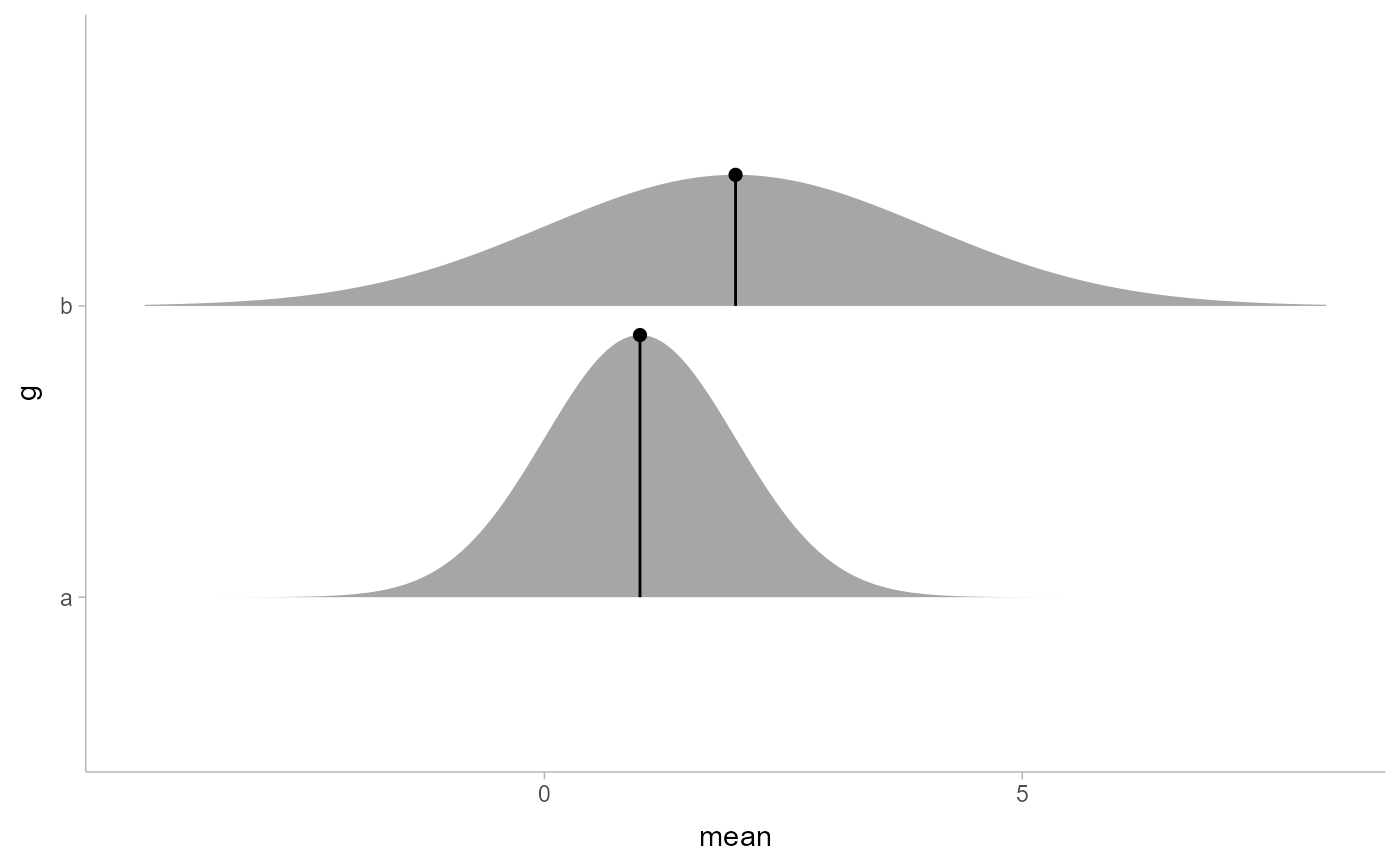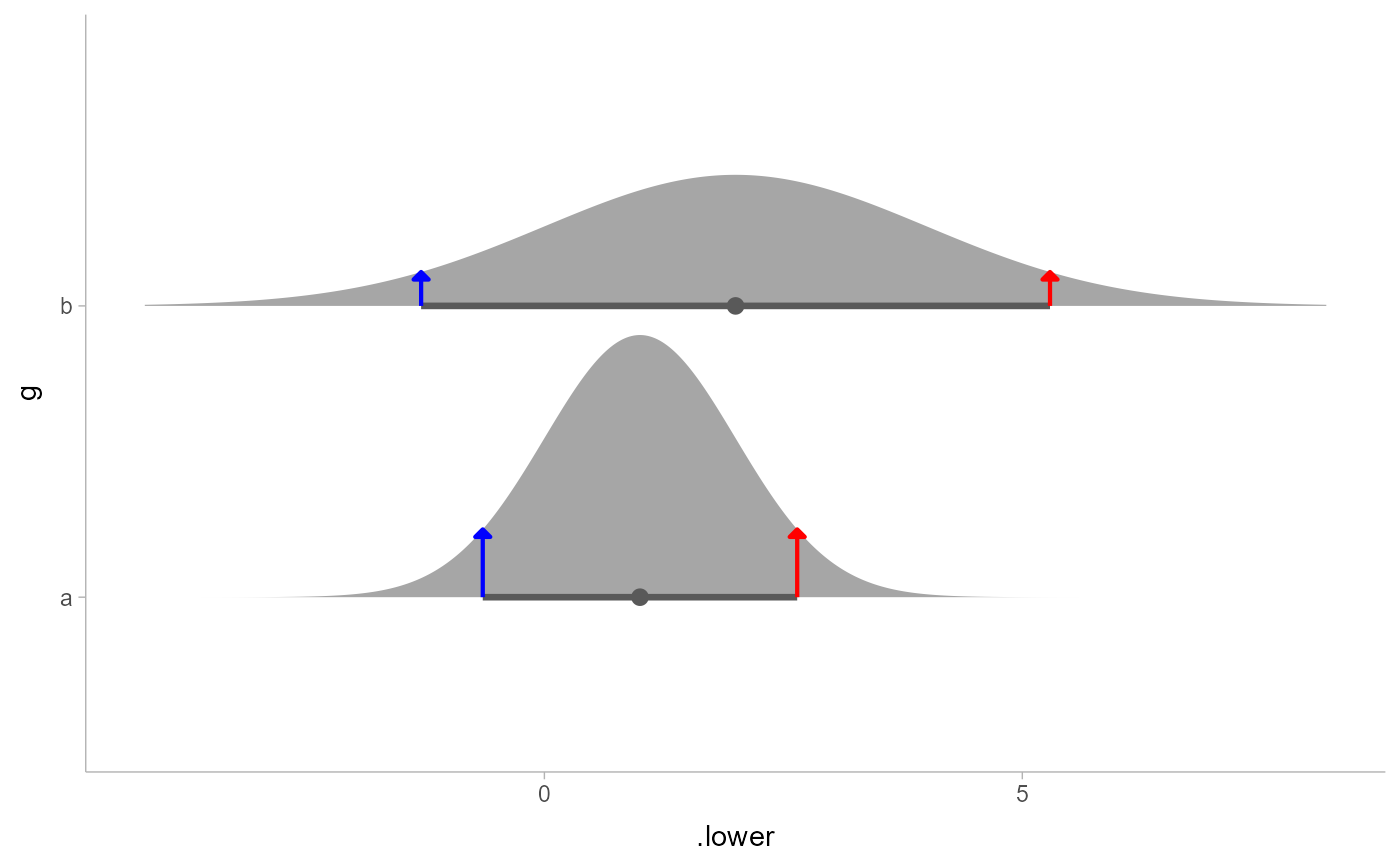Geometry for drawing "spikes" (optionally with points on them) on top of geom_slabinterval() geometries: this geometry understands the scaling and positioning of the thickness aesthetic from geom_slabinterval(), which allows you to position spikes and points along a slab.

geom_spike(
mapping = NULL,
data = NULL,
stat = "identity",
position = "identity",
...,
arrow = NULL,
orientation = NA,
normalize = "all",
na.rm = FALSE,
show.legend = NA,
inherit.aes = TRUE
)

## Arguments

mapping

Set of aesthetic mappings created by aes(). If specified and inherit.aes = TRUE (the default), it is combined with the default mapping at the top level of the plot. You must supply mapping if there is no plot mapping.

data

The data to be displayed in this layer. There are three options:

If NULL, the default, the data is inherited from the plot data as specified in the call to ggplot().

A data.frame, or other object, will override the plot data. All objects will be fortified to produce a data frame. See fortify() for which variables will be created.

A function will be called with a single argument, the plot data. The return value must be a data.frame, and will be used as the layer data. A function can be created from a formula (e.g. ~ head(.x, 10)).

stat

The statistical transformation to use on the data for this layer, either as a ggproto Geom subclass or as a string naming the stat stripped of the stat_ prefix (e.g. "count" rather than "stat_count")

position

Position adjustment, either as a string, or the result of a call to a position adjustment function. Setting this equal to "dodge" (position_dodge()) or "dodgejust" (position_dodgejust()) can be useful if you have overlapping geometries.

...

Other arguments passed to layer(). These are often aesthetics, used to set an aesthetic to a fixed value, like colour = "red" or linewidth = 3 (see Aesthetics, below). They may also be parameters to the paired geom/stat.

arrow

grid::arrow() giving the arrow heads to use on the spike, or NULL for no arrows.

orientation

Whether this geom is drawn horizontally or vertically. One of:

• NA (default): automatically detect the orientation based on how the aesthetics are assigned. Automatic detection works most of the time.

• "horizontal" (or "y"): draw horizontally, using the y aesthetic to identify different groups. For each group, uses the x, xmin, xmax, and thickness aesthetics to draw points, intervals, and slabs.

• "vertical" (or "x"): draw vertically, using the x aesthetic to identify different groups. For each group, uses the y, ymin, ymax, and thickness aesthetics to draw points, intervals, and slabs.

For compatibility with the base ggplot naming scheme for orientation, "x" can be used as an alias for "vertical" and "y" as an alias for "horizontal" (ggdist had an orientation parameter before base ggplot did, hence the discrepancy).

normalize

How to normalize heights of functions input to the thickness aesthetic. One of:

• "all": normalize so that the maximum height across all data is 1.

• "panels": normalize within panels so that the maximum height in each panel is 1.

• "xy": normalize within the x/y axis opposite the orientation of this geom so that the maximum height at each value of the opposite axis is 1.

• "groups": normalize within values of the opposite axis and within each group so that the maximum height in each group is 1.

• "none": values are taken as is with no normalization (this should probably only be used with functions whose values are in [0,1], such as CDFs).

na.rm

If FALSE, the default, missing values are removed with a warning. If TRUE, missing values are silently removed.

show.legend

logical. Should this layer be included in the legends? NA, the default, includes if any aesthetics are mapped. FALSE never includes, and TRUE always includes. It can also be a named logical vector to finely select the aesthetics to display.

inherit.aes

If FALSE, overrides the default aesthetics, rather than combining with them. This is most useful for helper functions that define both data and aesthetics and shouldn't inherit behaviour from the default plot specification, e.g. borders().

## Value

A ggplot2::Geom representing a spike geometry which can be added to a ggplot() object. rd_slabinterval_aesthetics(geom_name),

## Details

This geometry consists of a "spike" (vertical/horizontal line segment) and a "point" (at the end of the line segment). It uses the thickness aesthetic to determine where the endpoint of the line is, which allows it to be used with geom_slabinterval() geometries for labeling specific values of the thickness function.

## Aesthetics

The spike geom has a wide variety of aesthetics that control the appearance of its two sub-geometries: the spike and the point.

Positional aesthetics

• x: x position of the geometry

• y: y position of the geometry

Spike-specific (aka Slab-specific) aesthetics

• thickness: The thickness of the slab at each x value (if orientation = "horizontal") or y value (if orientation = "vertical") of the slab.

• side: Which side to place the slab on. "topright", "top", and "right" are synonyms which cause the slab to be drawn on the top or the right depending on if orientation is "horizontal" or "vertical". "bottomleft", "bottom", and "left" are synonyms which cause the slab to be drawn on the bottom or the left depending on if orientation is "horizontal" or "vertical". "topleft" causes the slab to be drawn on the top or the left, and "bottomright" causes the slab to be drawn on the bottom or the right. "both" draws the slab mirrored on both sides (as in a violin plot).

• scale: What proportion of the region allocated to this geom to use to draw the slab. If scale = 1, slabs that use the maximum range will just touch each other. Default is 0.9 to leave some space.

Color aesthetics

• colour: (or color) The color of the spike and point sub-geometries.

• fill: The fill color of the point sub-geometry.

• alpha: The opacity of the spike and point sub-geometries.

• colour_ramp: (or color_ramp) A secondary scale that modifies the color scale to "ramp" to another color. See scale_colour_ramp() for examples.

• fill_ramp: A secondary scale that modifies the fill scale to "ramp" to another color. See scale_fill_ramp() for examples.

Line aesthetics

• linewidth: Width of the line used to draw the spike sub-geometry.

• size: Size of the point sub-geometry.

• stroke: Width of the outline around the point sub-geometry.

• linetype: Type of line (e.g., "solid", "dashed", etc) used to draw the spike.

Other aesthetics (these work as in standard geoms)

• width

• height

• group

See examples of some of these aesthetics in action in vignette("slabinterval"). Learn more about the sub-geom override aesthetics (like interval_color) in the scales documentation. Learn more about basic ggplot aesthetics in vignette("ggplot2-specs").

See stat_spike() for the stat version, intended for use on sample data or analytical distributions.

Other slabinterval geoms: geom_interval(), geom_pointinterval(), geom_slab()

## Examples

library(ggplot2)
library(distributional)
library(dplyr)

# geom_spike is easiest to use with distributional or
# posterior::rvar objects
df = tibble(
d = dist_normal(1:2, 1:2), g = c("a", "b")
)

# annotate the density at the mean of a distribution
df %>% mutate(
mean = mean(d),
density(d, list(density_at_mean = mean))
) %>%
ggplot(aes(y = g)) +
stat_slab(aes(xdist = d)) +
geom_spike(aes(x = mean, thickness = density_at_mean)) +
# need shared thickness scale so that stat_slab and geom_spike line up
scale_thickness_shared()# annotate the endpoints of intervals of a distribution
# here we'll use an arrow instead of a point by setting size = 0
arrow_spec = arrow(angle = 45, type = "closed", length = unit(4, "pt"))
df %>% mutate(
median_qi(d, .width = 0.9),
density(d, list(density_lower = .lower, density_upper = .upper))
) %>%
ggplot(aes(y = g)) +
stat_halfeye(aes(xdist = d), .width = 0.9, color = "gray35") +
geom_spike(
aes(x = .lower, thickness = density_lower),
size = 0, arrow = arrow_spec, color = "blue", linewidth = 0.75
) +
geom_spike(
aes(x = .upper, thickness = density_upper),
size = 0, arrow = arrow_spec, color = "red", linewidth = 0.75
) +
scale_thickness_shared()